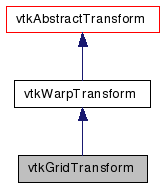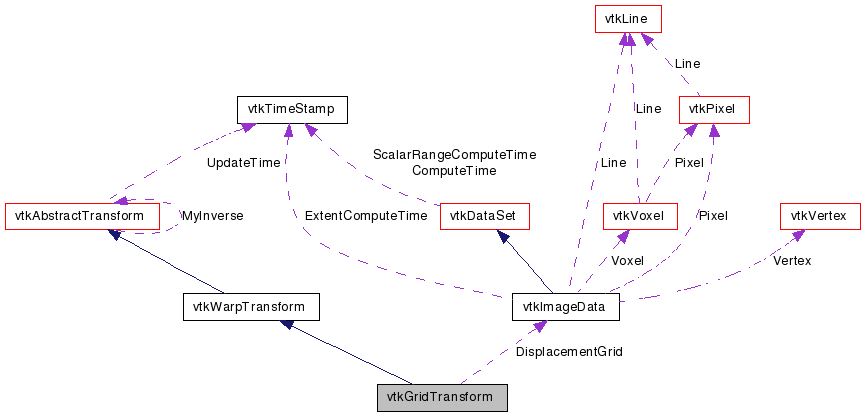# vtkGridTransform Class Reference

`#include <vtkGridTransform.h>`

Inheritance diagram for vtkGridTransform:[legend]
Collaboration diagram for vtkGridTransform:[legend]

## Detailed Description

a nonlinear warp transformation

vtkGridTransform describes a nonlinear warp transformation as a set of displacement vectors sampled along a uniform 3D grid.

Warning:
The inverse grid transform is calculated using an iterative method, and is several times more expensive than the forward transform.
vtkThinPlateSplineTransform vtkGeneralTransform vtkTransformToGrid
Tests:
vtkGridTransform (Tests)

Definition at line 41 of file vtkGridTransform.h.

## Public Types

typedef vtkWarpTransform Superclass

## Public Member Functions

virtual const char * GetClassName ()
virtual int IsA (const char *type)
virtual void PrintSelf (ostream &os, vtkIndent indent)
vtkAbstractTransformMakeTransform ()
unsigned long GetMTime ()
virtual void SetDisplacementGrid (vtkImageData *)
virtual vtkImageDataGetDisplacementGrid ()
virtual void SetDisplacementScale (double)
virtual double GetDisplacementScale ()
virtual void SetDisplacementShift (double)
virtual double GetDisplacementShift ()
void SetInterpolationMode (int mode)
virtual int GetInterpolationMode ()
void SetInterpolationModeToNearestNeighbor ()
void SetInterpolationModeToLinear ()
void SetInterpolationModeToCubic ()
const char * GetInterpolationModeAsString ()

## Static Public Member Functions

static vtkGridTransformNew ()
static int IsTypeOf (const char *type)
static vtkGridTransformSafeDownCast (vtkObject *o)

## Protected Member Functions

vtkGridTransform ()
~vtkGridTransform ()
void InternalUpdate ()
void InternalDeepCopy (vtkAbstractTransform *transform)
void ForwardTransformDerivative (const float in, float out, float derivative)
void ForwardTransformDerivative (const double in, double out, double derivative)
void InverseTransformPoint (const float in, float out)
void InverseTransformPoint (const double in, double out)
void InverseTransformDerivative (const float in, float out, float derivative)
void InverseTransformDerivative (const double in, double out, double derivative)
void ForwardTransformPoint (const float in, float out)
void ForwardTransformPoint (const double in, double out)

## Protected Attributes

void(* InterpolationFunction )(double point, double displacement, double derivatives, void *gridPtr, int gridType, int inExt, vtkIdType inInc)
int InterpolationMode
double DisplacementScale
double DisplacementShift
void * GridPointer
int GridScalarType
double GridSpacing 
double GridOrigin 
int GridExtent 
vtkIdType GridIncrements 

## Member Typedef Documentation

Reimplemented from vtkWarpTransform.

Definition at line 45 of file vtkGridTransform.h.

## Constructor & Destructor Documentation

 vtkGridTransform::vtkGridTransform ( ) ` [protected]`

 vtkGridTransform::~vtkGridTransform ( ) ` [protected]`

## Member Function Documentation

 static vtkGridTransform* vtkGridTransform::New ( ) ` [static]`

Create an object with Debug turned off, modified time initialized to zero, and reference counting on.

Reimplemented from vtkObject.

 virtual const char* vtkGridTransform::GetClassName ( ) ` [virtual]`

Reimplemented from vtkWarpTransform.

 static int vtkGridTransform::IsTypeOf ( const char * name ) ` [static]`

Return 1 if this class type is the same type of (or a subclass of) the named class. Returns 0 otherwise. This method works in combination with vtkTypeMacro found in vtkSetGet.h.

Reimplemented from vtkWarpTransform.

 virtual int vtkGridTransform::IsA ( const char * name ) ` [virtual]`

Return 1 if this class is the same type of (or a subclass of) the named class. Returns 0 otherwise. This method works in combination with vtkTypeMacro found in vtkSetGet.h.

Reimplemented from vtkWarpTransform.

 static vtkGridTransform* vtkGridTransform::SafeDownCast ( vtkObject * o ) ` [static]`

Reimplemented from vtkWarpTransform.

 virtual void vtkGridTransform::PrintSelf ( ostream & os, vtkIndent indent ) ` [virtual]`

Methods invoked by print to print information about the object including superclasses. Typically not called by the user (use Print() instead) but used in the hierarchical print process to combine the output of several classes.

Reimplemented from vtkWarpTransform.

 virtual void vtkGridTransform::SetDisplacementGrid ( vtkImageData * ) ` [virtual]`

Set/Get the grid transform (the grid transform must have three components for displacement in x, y, and z respectively). The vtkGridTransform class will never modify the data.

 virtual vtkImageData* vtkGridTransform::GetDisplacementGrid ( ) ` [virtual]`

Set/Get the grid transform (the grid transform must have three components for displacement in x, y, and z respectively). The vtkGridTransform class will never modify the data.

 virtual void vtkGridTransform::SetDisplacementScale ( double ) ` [virtual]`

Set scale factor to be applied to the displacements. This is used primarily for grids which contain integer data types. Default: 1

 virtual double vtkGridTransform::GetDisplacementScale ( ) ` [virtual]`

Set scale factor to be applied to the displacements. This is used primarily for grids which contain integer data types. Default: 1

 virtual void vtkGridTransform::SetDisplacementShift ( double ) ` [virtual]`

Set a shift to be applied to the displacements. The shift is applied after the scale, i.e. x = scale*y + shift. Default: 0

 virtual double vtkGridTransform::GetDisplacementShift ( ) ` [virtual]`

Set a shift to be applied to the displacements. The shift is applied after the scale, i.e. x = scale*y + shift. Default: 0

 void vtkGridTransform::SetInterpolationMode ( int mode )

Set interpolation mode for sampling the grid. Higher-order interpolation allows you to use a sparser grid. Default: Linear.

 virtual int vtkGridTransform::GetInterpolationMode ( ) ` [virtual]`

Set interpolation mode for sampling the grid. Higher-order interpolation allows you to use a sparser grid. Default: Linear.

 void vtkGridTransform::SetInterpolationModeToNearestNeighbor ( ) ` [inline]`

Set interpolation mode for sampling the grid. Higher-order interpolation allows you to use a sparser grid. Default: Linear.

Definition at line 75 of file vtkGridTransform.h.

 void vtkGridTransform::SetInterpolationModeToLinear ( ) ` [inline]`

Set interpolation mode for sampling the grid. Higher-order interpolation allows you to use a sparser grid. Default: Linear.

Definition at line 77 of file vtkGridTransform.h.

 void vtkGridTransform::SetInterpolationModeToCubic ( ) ` [inline]`

Set interpolation mode for sampling the grid. Higher-order interpolation allows you to use a sparser grid. Default: Linear.

Definition at line 79 of file vtkGridTransform.h.

 const char * vtkGridTransform::GetInterpolationModeAsString ( ) ` [inline]`

Set interpolation mode for sampling the grid. Higher-order interpolation allows you to use a sparser grid. Default: Linear.

Definition at line 145 of file vtkGridTransform.h.

 vtkAbstractTransform* vtkGridTransform::MakeTransform ( ) ` [virtual]`

Make another transform of the same type.

Implements vtkAbstractTransform.

 unsigned long vtkGridTransform::GetMTime ( ) ` [virtual]`

Get the MTime.

Reimplemented from vtkAbstractTransform.

 void vtkGridTransform::InternalUpdate ( ) ` [protected, virtual]`

Update the displacement grid.

Reimplemented from vtkAbstractTransform.

 void vtkGridTransform::InternalDeepCopy ( vtkAbstractTransform * transform ) ` [protected, virtual]`

Copy this transform from another of the same type.

Reimplemented from vtkAbstractTransform.

 void vtkGridTransform::ForwardTransformPoint ( const float in, float out ) ` [protected, virtual]`

Internal functions for calculating the transformation.

Implements vtkWarpTransform.

 void vtkGridTransform::ForwardTransformPoint ( const double in, double out ) ` [protected, virtual]`

Internal functions for calculating the transformation.

Implements vtkWarpTransform.

 void vtkGridTransform::ForwardTransformDerivative ( const float in, float out, float derivative ) ` [protected, virtual]`

Calculate the forward transform as well as the derivative.

Implements vtkWarpTransform.

 void vtkGridTransform::ForwardTransformDerivative ( const double in, double out, double derivative ) ` [protected, virtual]`

Calculate the forward transform as well as the derivative.

Implements vtkWarpTransform.

 void vtkGridTransform::InverseTransformPoint ( const float in, float out ) ` [protected, virtual]`

If the InverseFlag is set to 1, then a call to InternalTransformPoint results in a call to InverseTransformPoint. The inverse transformation is calculated from using Newton's method.

Reimplemented from vtkWarpTransform.

 void vtkGridTransform::InverseTransformPoint ( const double in, double out ) ` [protected, virtual]`

If the InverseFlag is set to 1, then a call to InternalTransformPoint results in a call to InverseTransformPoint. The inverse transformation is calculated from using Newton's method.

Reimplemented from vtkWarpTransform.

 void vtkGridTransform::InverseTransformDerivative ( const float in, float out, float derivative ) ` [protected, virtual]`

Calculate the inverse transform as well as the derivative of the forward transform (that's correct: the derivative of the forward transform, not of the inverse transform)

Reimplemented from vtkWarpTransform.

 void vtkGridTransform::InverseTransformDerivative ( const double in, double out, double derivative ) ` [protected, virtual]`

Calculate the inverse transform as well as the derivative of the forward transform (that's correct: the derivative of the forward transform, not of the inverse transform)

Reimplemented from vtkWarpTransform.

## Member Data Documentation

 void(* vtkGridTransform::InterpolationFunction)(double point, double displacement, double derivatives, void *gridPtr, int gridType, int inExt, vtkIdType inInc)` [protected]`

 int vtkGridTransform::InterpolationMode` [protected]`

Definition at line 125 of file vtkGridTransform.h.

 vtkImageData* vtkGridTransform::DisplacementGrid` [protected]`

Definition at line 126 of file vtkGridTransform.h.

 double vtkGridTransform::DisplacementScale` [protected]`

Definition at line 127 of file vtkGridTransform.h.

 double vtkGridTransform::DisplacementShift` [protected]`

Definition at line 128 of file vtkGridTransform.h.

 void* vtkGridTransform::GridPointer` [protected]`

Definition at line 130 of file vtkGridTransform.h.

 int vtkGridTransform::GridScalarType` [protected]`

Definition at line 131 of file vtkGridTransform.h.

 double vtkGridTransform::GridSpacing` [protected]`

Definition at line 132 of file vtkGridTransform.h.

 double vtkGridTransform::GridOrigin` [protected]`

Definition at line 133 of file vtkGridTransform.h.

 int vtkGridTransform::GridExtent` [protected]`

Definition at line 134 of file vtkGridTransform.h.

 vtkIdType vtkGridTransform::GridIncrements` [protected]`

Definition at line 135 of file vtkGridTransform.h.

The documentation for this class was generated from the following file:

Generated on Wed Aug 24 11:39:04 2011 for VTK by1.5.6